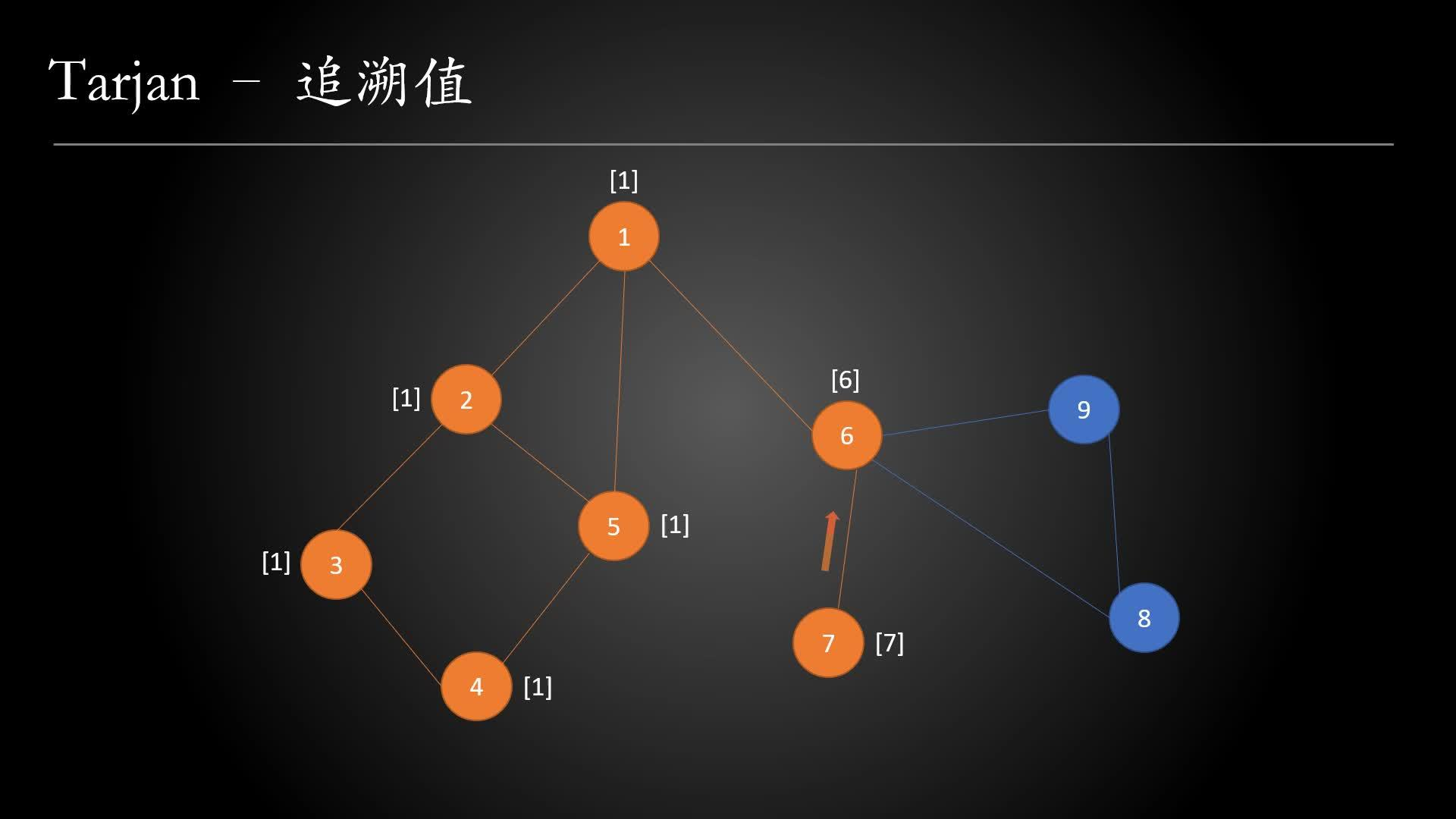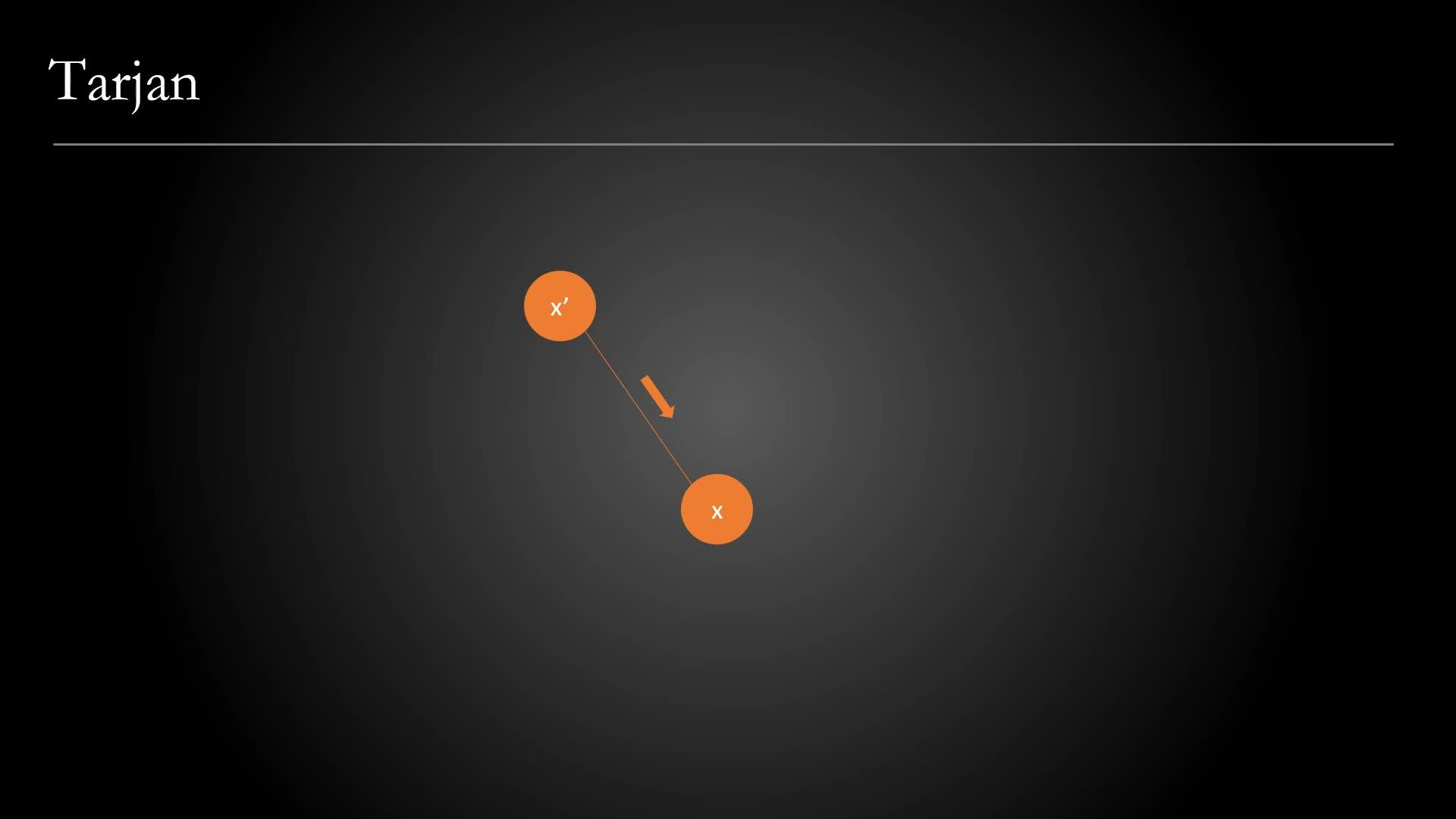Tarjan 算法是图论中非常实用 / 常用的算法之一，能解决强连通分量，双连通分量，割点和桥，求最近公共祖先（LCA）等问题。

## 一、Tarjan 算法

Tarjan 算法是基于深度优先搜索的算法，用于求解图的连通性问题。Tarjan 算法可以在线性时间内求出无向图的割点与桥，进一步地可以求解无向图的双连通分量；同时，也可以求解有向图的强连通分量、必经点与必经边。

## 时间戳

​时间戳是用来标记图中每个节点在进行深度优先搜索时被访问的时间顺序，当然，你可以理解成一个序号（这个序号由小到大），用 dfn[x] 来表示。

## 追溯值

• 以 x 为根的搜索树的所有节点
• 通过一条非搜索树上的边，能够到达搜索树的所有节点https://www.zhihu.com/video/1198570627823767552

## 无向图的桥判定法则

• 以节点 v 为根的搜索树中的所有节点
• 通过一条非搜索树上的边，能够到达搜索树的所有节点（在追溯值内容中有所解释）

## C++ 实现

``````// tarjan 算法求无向图的桥
#include<iostream>
#include<cstdio>
#include<cstring>
#include<algorithm>
#include<vector>
​
using namespace std;
​
const int SIZE = 100010;
int head[SIZE], ver[SIZE * 2], Next[SIZE * 2];
int dfn[SIZE], low[SIZE];
int n, m, tot, num;
bool bridge[SIZE * 2];
​
void add(int x, int y) {
}
​
void tarjan(int x, int in_edge) {
dfn[x] = low[x] = ++num;
for (int i = head[x]; i; i = Next[i]) {
int y = ver[i];
if (!dfn[y]) {
tarjan(y, i);
low[x] = min(low[x], low[y]);
if (low[y] > dfn[x])
bridge[i] = bridge[i ^ 1] = true;
}
else if (i != (in_edge ^ 1))
low[x] = min(low[x], dfn[y]);
}
}
​
int main() {
// [[0,1],[1,2],[2,0],[1,3]]
cin >> n >> m;
tot = 1;
for (int i = 1; i <= m; i++) {
int x, y;
scanf("%d%d", &x, &y);
}
for (int i = 1; i <= n; i++)
if (!dfn[i]) tarjan(i, 0);
for (int i = 2; i < tot; i += 2)
if (bridge[i])
printf("%d %d\n", ver[i ^ 1], ver[i]);
}
``````

``````const int SIZE = 100010;
int head[SIZE], ver[SIZE * 2], Next[SIZE * 2];
int dfn[SIZE], low[SIZE];
int n, m, tot, num;
bool bridge[SIZE * 2];
``````
• SIZE —— 节点的个数，可以基于实际的节点个数增加一些冗余度
• 关于 head / Next / ver 变量的介绍，可以参阅下面的动画配合理解
• 假设场景，有一个节点 u，以及其直接相连的若干节点 v1，v2，v3
• head[u] 代表节点 u 直接相邻的第一个节点 v1 的序号（tot1）
• Next[tot1] 代表节点 u 直接相邻的下一个节点的序号（tot2）
• ver[tot1] 代表序号为 tot1 的节点的值（v1）
• dfn[x] 代表节点 x 对应的时间戳，low[x] 代表节点 x 的追溯值
• n 与 m 代表程序的标准输入的参数，n 代表节点的个数，m 代表边的个数
• tot 代表每一个节点的序号
• num 代表每一个节点的时间戳
• bridge[tot] 代表序号为 tot 的边是否为桥

``````// x 代表当前搜索树的根节点，in_edge 代表其对应的序号（tot）
void tarjan(int x, int in_edge) {
// 在搜索之前，先初始化节点 x 的时间戳与追溯值
dfn[x] = low[x] = ++num;
// 通过 head 变量获取节点 x 的直接连接的第一个相邻节点的序号
// 通过 Next 变量，迭代获取剩下的与节点 x 直接连接的节点的序号
for (int i = head[x]; i; i = Next[i]) {
// 此时，i 代表节点 y 的序号
int y = ver[i];
// 如果当前节点 y 没有被访问过
if (!dfn[y]) {
// 递归搜索以 y 为跟的子树
tarjan(y, i);
// 计算 x 的追溯值
low[x] = min(low[x], low[y]);
// 桥的判定法则
if (low[y] > dfn[x])
bridge[i] = bridge[i ^ 1] = true; // 标记当前节点是否为桥（具体见下文）
}
else if (i != (in_edge ^ 1)) // 当前节点被访问过，且 y 不是 x 的“父节点”（具体见下文）
low[x] = min(low[x], dfn[y]);
}
}
``````

## 五、语句详解

### 语句一：i != (in_edge ^ 1)https://www.zhihu.com/video/1198571871527161856

• 如果 x 为偶数（2），那么 x ^ 1 = 2 ^ 1 = 3
• 如果 x 为奇数（3），那么 x ^ 1 = 3 ^ 1 = 2

## ​七、实践题

「关键连接」是在该集群中的重要连接，也就是说，假如我们将它移除，便会导致某些服务器无法访问其他服务器。

``````输入：n = 4, connections = [[0,1],[1,2],[2,0],[1,3]]

​提示

• `1 <= n <= 10^5`
• `n-1 <= connections.length <= 10^5`
• `connections[i] != connections[i]`
• 不存在重复的连接